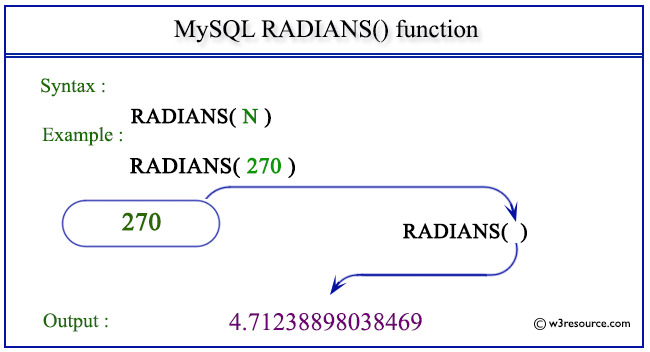MySQL RADIANS() converts the value of a number from degrees to radians. (pi radians equals to 180 degrees).

Syntax:

```RADIANS(M);
```

Argument

Name Description
M A number in degrees which is to be converted to radians.

Syntax Diagram:MySQL Version: 5.6

Pictorial presentation of MySQL RADIANS() functionCode:

``````SELECT RADIANS(270);
```
```

Explanation

The above MySQL statement converts 270 (in degrees) to radians.

Sample Output:

```mysql> SELECT RADIANS(270);
+------------------+
+------------------+
| 4.71238898038469 |
+------------------+
1 row in set (0.00 sec)
```

All Mathematical FunctionsPrevious: POWER()
Next: RAND()

﻿